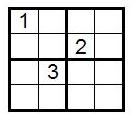### Contents

Home

1. Introduction to Sudoku

2. Solving Strategy

3. Counting Solutions

4. Solution Symmetries

5. The 4×4 Case

6. Some More Interesting Facts

7. References

# The Math Behind Sudoku

## Some More Interesting Facts

A well-formed Sudoku puzzle is one that has a unique solution. A Sudoku puzzle can have more than one solution, but in this case the kind of logical reasoning we described while discussing solving strategies may fall short. There are examples of rank-3 Sudoku puzzles with 17 givens that are well-formed. However, the minimum number of givens for which a rank-3 Sudoku can be well-formed is not known.

Exercise: Can you come up with a Sudoku puzzle that is not well-formed?

Another interesting question (that you may have considered when solving the above exercise) is how many distinct symbols need to be used among the givens for a puzzle to be well-formed? It turns out that for a Sudoku of rank n, at least n2-1 distinct symbols must be used for the puzzle to have a unique solution. This is because if we had a rank-n puzzle where only n2-2 symbols were used and we found a solution, then interchanging the places of the two symbols missing from the givens would result in another, different solution. In particular, for the usual rank-3 Sudoku, at least 32-1=8 distinct digits must be used in the givens for the puzzle to be well-formed; otherwise, the puzzle will have more than one solution.

The fact discussed above can be restated as follows: If a Sudoku of rank n is well-formed, then it must have n2-1 distinct digits among the givens. It is important to note that this is not the same as stating that if a Sudoku of rank n has n2-1 distinct digits in the givens, then it is well-formed. Recall that the converse of a true statement is not necessarily true. The next exercise illustrates this with a specific example.

Exercise: The following rank-2 Sudoku has 22-1=3 distinct digits among the givens. Find two different solutions.For 4×4 Sudoku, a case-by-case analysis utilizing the two essentially different grids proves that a well-formed puzzle must have a minimum of four distinct digits in the givens.

Finally, it is intriguing to note that even though there are computer programs that can quickly and easily solve rank-3 Sudokus by employing a backtracking method, solving a Sudoku of arbitrary rank n is a much more difficult problem. As the rank of a Sudoku increases from n to n+1, the extra computational time needed to find a solution increases quite fast. This places the game of solving rank-n Sudoku puzzles in a class of problems that computer scientists have named NP-complete. An NP-complete problem satisfies the following two properties:

1. Any solution to the problem can be checked relatively quickly, i.e. in polynomial time.
2. If the problem can be solved relatively quickly, then so can every problem that satisfies property (1).
Even though checking a solution of an NP-complete problem can be done relatively quickly and easily, there is no known algorithm for finding a solution to begin with, because the time needed for computation increases so fast compared to the size of the problem.